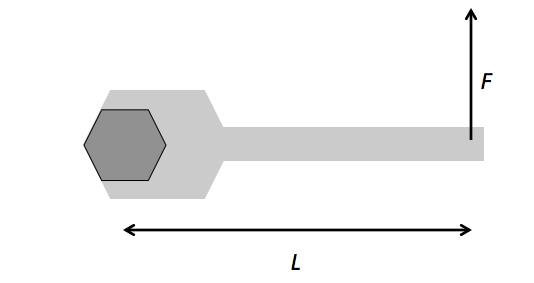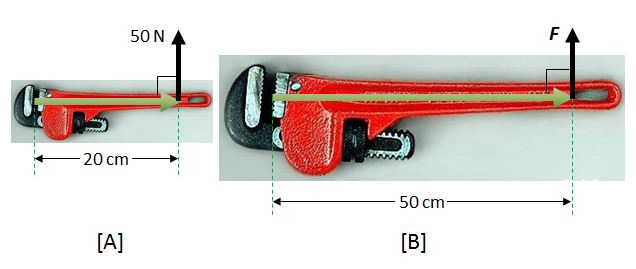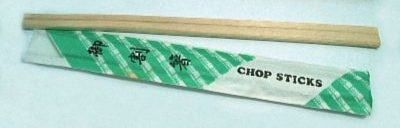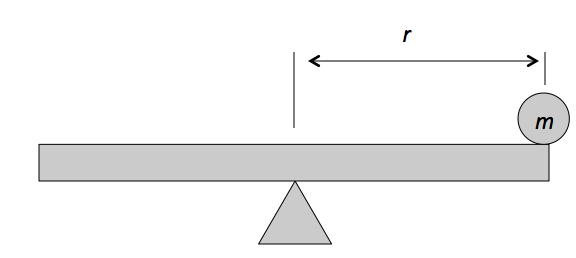Classical Mechanics

# Torque - rotational form of Newton's second lawIn an effort to loosen a bolt, a force of $$F = 200 \text{ N}$$ that is perpendicular to the wrench is applied, as shown in the figure above. If the distance from the end of the wrench to the center of the bolt is $$L = 26 \text{ cm},$$ what is the magnitude of the torque produced by $$F?$$The picture [A] above is a $$20$$ cm wrench which can tighten a bolt with at least $$50$$ N of force. If the length of the wrench is increased to $$50$$ cm as in [B] above, what is minimum force required for it to tighten the same bolt as before? (The forces are acting vertical to the axis of the wrench in both cases.)Jake is on vacation in Japan (for real!), preparing to enjoy Japanese cuisine when he realizes that breaking chopsticks from the end is far easier than from the middle.

What concept explains this?A mass $$m = 8 \text{ kg}$$ is gently placed on one end of a see-saw. If the distance between the pivot point of the see-saw and the mass $$m$$ is $$r= 1 \text{ m} ,$$ what is the magnitude of torque on the mass?

The gravitational acceleration is $$g= 10 \text{ m/s}^2.$$

We use a wrench to turn nuts on bolts because they require less force. Consider a hexagonal nut $$1~\mbox{cm}$$ in diameter. We can tighten this nut with one of two wrenches, wrench A with lever arm $$10~\mbox{cm}$$ and wrench B with lever arm $$20~\mbox{cm}$$. Both wrenches have a very small mass, so you may neglect their masses in this problem. What is the ratio of the total work it would take to tighten the nut one full turn with wrench A to the total work it would take with wrench B?

×